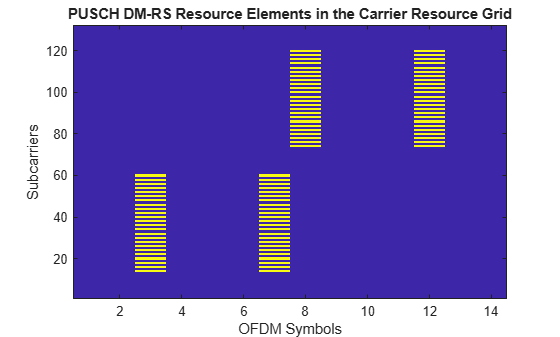# nrPUSCHDMRSIndices

Generate PUSCH DM-RS indices

## Syntax

``ind = nrPUSCHDMRSIndices(carrier,pusch)``
``ind = nrPUSCHDMRSIndices(carrier,pusch,Name,Value)``

## Description

example

````ind = nrPUSCHDMRSIndices(carrier,pusch)` returns a matrix containing demodulation reference signal (DM-RS) resource element (RE) indices of a physical uplink shared channel (PUSCH), as defined in TS 38.211 Section 6.4.1.1.3 . `carrier` specifies the carrier configuration parameters for a specific OFDM numerology. `pusch` specifies the PUSCH configuration parameters. The returned indices are 1-based using linear indexing form.```

example

````ind = nrPUSCHDMRSIndices(carrier,pusch,Name,Value)` specifies output formatting options by using one or more name-value pair arguments. Unspecified options take default values.```

## Examples

collapse all

Create a carrier configuration object with default properties. This object corresponds to a 10 MHz carrier with 15 kHz subcarrier spacing.

`carrier = nrCarrierConfig;`

Create a physical uplink shared channel (PUSCH) configuration object with specified properties. When transform precoding is set to 0, the waveform type is cyclic-prefix orthogonal frequency division multiplexing (CP-OFDM).

```pusch = nrPUSCHConfig; pusch.TransformPrecoding = 0; pusch.TransmissionScheme = 'codebook'; pusch.NumAntennaPorts = 4; pusch.TPMI = 0;```

Configure PUSCH demodulation reference signal (DM-RS) object with specified parameters.

```pusch.DMRS.DMRSAdditionalPosition = 2; pusch.DMRS.DMRSTypeAPosition = 2; pusch.DMRS.DMRSPortSet = 3; pusch.DMRS.NIDNSCID = 15; pusch.DMRS.NSCID = 1;```

Generate DM-RS indices associated to PUSCH of subscript indexing form.

`ind = nrPUSCHDMRSIndices(carrier,pusch,'IndexStyle','subscript')`
```ind = 3744x3 uint32 matrix 2 3 1 4 3 1 6 3 1 8 3 1 10 3 1 12 3 1 14 3 1 16 3 1 18 3 1 20 3 1 ⋮ ```

Create a carrier configuration with 30 kHz subcarrier spacing and 5 MHz transmission bandwidth.

```carrier = nrCarrierConfig; carrier.SubcarrierSpacing = 30; carrier.NSizeGrid = 11;```

Create a physical uplink shared channel (PUSCH) configuration object with specified properties. When transform precoding is set to 1, the waveform type is discrete fourier transform spread orthogonal frequency division multiplexing (DFT-s-OFDM).

```pusch = nrPUSCHConfig; pusch.NSizeBWP = 9; pusch.NStartBWP = 1; pusch.PRBSet = 0:3; pusch.TransformPrecoding = 1; pusch.FrequencyHopping = 'intraSlot'; pusch.SecondHopStartPRB = 5;```

Create a PUSCH demodulation reference signal (DM-RS) object with specified properties.

```dmrs = nrPUSCHDMRSConfig; dmrs.DMRSAdditionalPosition = 1; dmrs.DMRSTypeAPosition = 2; dmrs.DMRSPortSet = 3; dmrs.GroupHopping = 1; dmrs.SequenceHopping = 0; dmrs.NRSID = 10;```

Assign the PUSCH DM-RS configuration object to DMRS property of PUSCH configuration object.

`pusch.DMRS = dmrs;`

Generate PUSCH DM-RS symbols and indices for the specified carrier, PUSCH configuration, and output formatting `name-value` pair argument.

`sym = nrPUSCHDMRS(carrier,pusch,'OutputDataType','single')`
```sym = 96x1 single column vector -0.7071 - 0.7071i -0.7071 - 0.7071i -0.7071 - 0.7071i 0.7071 - 0.7071i -0.7071 - 0.7071i -0.7071 - 0.7071i -0.7071 - 0.7071i 0.7071 - 0.7071i 0.7071 + 0.7071i -0.7071 + 0.7071i ⋮ ```
`ind = nrPUSCHDMRSIndices(carrier,pusch,'IndexBase','0based','IndexOrientation','bwp')`
```ind = 96x1 uint32 column vector 217 219 221 223 225 227 229 231 233 235 ⋮ ```

Create a bandwidth part (BWP) grid, and then map the DM-RS symbols on the grid.

```bwp = complex(zeros([pusch.NSizeBWP*12 carrier.SymbolsPerSlot pusch.NumLayers])); bwp(ind+1) = sym; % Map the DM-RS symbols```

Map the BWP to the carrier resource grid, and then display the carrier grid.

```grid = complex(zeros([carrier.NSizeGrid*12 carrier.SymbolsPerSlot pusch.NumLayers])); % Create carrier resource grid offset = pusch.NStartBWP-carrier.NStartGrid; % BWP start location in the carrier grid grid(offset*12+1:(offset+pusch.NSizeBWP)*12,:,:) = bwp; imagesc(abs(grid(:,:,1))); axis xy; xlabel('OFDM Symbols'); ylabel('Subcarriers'); title('PUSCH DM-RS Resource Elements in the Carrier Resource Grid');```## Input Arguments

collapse all

Carrier configuration parameters for a specific OFDM numerology, specified as an `nrCarrierConfig` object. This function uses only these properties of the `nrCarrierConfig` object.

PUSCH configuration parameters, specified as an `nrPUSCHConfig` object. This function uses only these properties of the `nrPUSCHConfig` object.

### Name-Value Arguments

Specify optional pairs of arguments as `Name1=Value1,...,NameN=ValueN`, where `Name` is the argument name and `Value` is the corresponding value. Name-value arguments must appear after other arguments, but the order of the pairs does not matter.

Before R2021a, use commas to separate each name and value, and enclose `Name` in quotes.

Example: `'IndexStyle','subscript','IndexBase','0based'` specifies nondefault resource element index formatting properties.

RE indexing form, specified as one of these values:

• `'index'` — The indices are in linear index form.

• `'subscript'` — The indices are in [subcarrier, symbol, antenna] subscript row form.

Data Types: `char` | `string`

RE indexing base, specified as one of these values:

• `'1based'` — The index counting starts from 1.

• `'0based'` — The index counting starts from 0.

Data Types: `char` | `string`

Indexing orientation of resource elements, specified as the comma-separated pair consisting of `'IndexOrientation'` and one of these values:

• `'carrier'` — Indices are referenced with respect to the carrier grid.

• `'bwp'` — Indices are referenced with respect to the bandwidth part.

Data Types: `char` | `string`

## Output Arguments

collapse all

DM-RS resource element indices, returned as one of these values:

• N-by-P matrix — The function returns this type of value when `'IndexStyle'` is set to `'index'`. The number of columns depends on the `TransmissionScheme` property of `nrPUSCHConfig` object and returned as one these values.

• Number of transmission layers — When the transmission scheme is non-codebook

• Number of antenna ports configured — When the transmission scheme is codebook

• M-by-3 matrix — The function returns this type of value when `'IndexStyle'` is set to `'subscript'`. The matrix rows correspond to the [subcarrier, symbol, antenna] subscripts based on the number of subcarriers, OFDM symbols, and number of antennas, respectively.

Depending on the value of `'IndexBase'`, the function returns either 1-based or 0-based indices. Depending on the value of `'IndexOrientation'`, the function returns either carrier oriented indices or BWP oriented indices.

Data Types: `uint32`

 3GPP TS 38.211. “NR; Physical channels and modulation.” 3rd Generation Partnership Project; Technical Specification Group Radio Access Network.